# Test: Optimal Power System Operation of Power Systems

## 10 Questions MCQ Test Power Systems | Test: Optimal Power System Operation of Power Systems

Description
Attempt Test: Optimal Power System Operation of Power Systems | 10 questions in 30 minutes | Mock test for Electrical Engineering (EE) preparation | Free important questions MCQ to study Power Systems for Electrical Engineering (EE) Exam | Download free PDF with solutions
QUESTION: 1

### If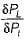for a plant i with output P1 and loss in the system being PL increases, the penalty factor of the system

Solution:

Penalty factor of the system is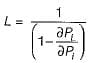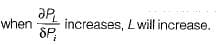QUESTION: 2

### The incremental fuel costs of two plants are given by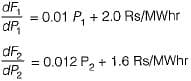For economic schedule of a load of 180 MW

Solution:

For economic scheduling of plants, we have: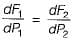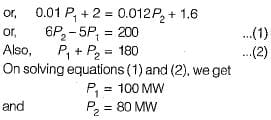QUESTION: 3

### A generator is supplying a load. An incremental change in load of 4 MW requires generation to be increased by 6 MW. The cost at plant bus is ₹ 30/MWhr. The incremental cost at the receiving end is

Solution: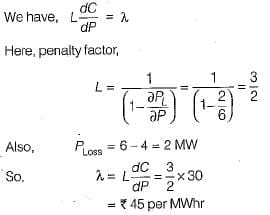QUESTION: 4

The current distribution factors for the power system network shown below are given by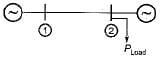Solution:

Since the load is at plant 2 therefore,
Na2 = 0 and Na1 = 1

QUESTION: 5

The incremental transmission loss of a plant can’t be a

Solution:

The incremental transmission loss of a plant can be positive, a non-zero value but, can’t be zero.

QUESTION: 6

When generating units are loaded to equal incremental costs, it results in

Solution:

To minimize the total fuel cost, the generating units must operate at equal incremental cost of production.

QUESTION: 7

Unit of heat rate curve is

Solution: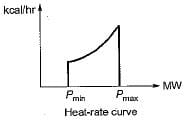QUESTION: 8

Unit of λ is

Solution: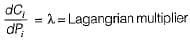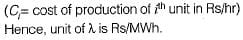QUESTION: 9

Cost curves are expressed as:

Solution:
QUESTION: 10

The optimization problem is

Solution:Use Code STAYHOME200 and get INR 200 additional OFF Use Coupon Code